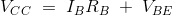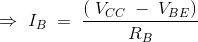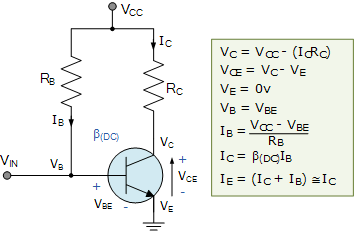# Bipolar Junction Transistor Fixed Base Biasing Electronics Engineering Notes 1st Year

## What is Transistor Biasing:

Transistor Biasing is the process of setting a transistors DC operating voltage or current conditions to the correct level so that any AC input signal can be amplified correctly by the transistor.

## Transistor Biasing:

Establishing the correct operating point requires the proper selection of bias resistors and load resistors to provide the appropriate input current and collector voltage conditions. The correct biasing point for a bipolar transistor, either NPN or PNP, generally lies somewhere between the two extremes of operation with respect to it being either “fully-ON” or “fully-OFF” along its load line. This central operating point is called the “Quiescent Operating Point”, or Q-point for short.

When a bipolar transistor is biased so that the Q-point is near the middle of its operating range, that is approximately halfway between cut-off and saturation, it is said to be operating as a Class-A amplifier.

This mode of operation allows the output current to increase and decrease around the amplifiers Q-point without distortion as the input signal swings through a complete cycle. In other words, the output current flows for the full 360o of the input cycle.

## Base Biasing a Common Emitter Amplifier:

One of the most frequently used biasing circuits for a transistor circuit is with the self-bias of the emitter-bias circuit where one or more biasing resistors are used to set up the initial DC values of transistor currents, ( IB ), ( IC ) and ( IE ).

## Fixed Base Biasing a Transistor:

In this condition, a single power source is applied to the collector and base of the transistor using only two resistors. Applying KVL to the circuit,Thus, by merely changing the value of the resistor the base current can be adjusted to the desired value. And by using the current gain (β) relationship, IC can also be found out accordingly. Hence the Q point can be adjusted just by changing the value of the resistor connected to the base.The circuit shown is called as a “fixed base bias circuit”, because the transistors base current, IB remains constant for given values of Vcc, and therefore the transistors operating point must also remain fixed. This two resistor biasing network is used to establish the initial operating region of the transistor using a fixed current bias.

This type of transistor biasing arrangement is also beta-dependent biasing as the steady-state condition of operation is a function of the transistors beta β value, so the biasing point will vary over a wide range for transistors of the same type as the characteristics of the transistors will not be exactly the same.

The emitter diode of the transistor is forward biased by applying the required positive base bias voltage via the current limiting resistor RB. Assuming a standard bipolar transistor, the forward base-emitter voltage drop will be 0.7V. Then the value of RB is simply: (VCC – VBE)/IB where IB is defined as IC.

With this single resistor type of biasing method the biasing voltages and currents do not remain stable during transistor operation and can vary enormously. Also the temperature of the transistor can adversely effect the operating point.

### Feedback is important to us.

error: Content is protected !!